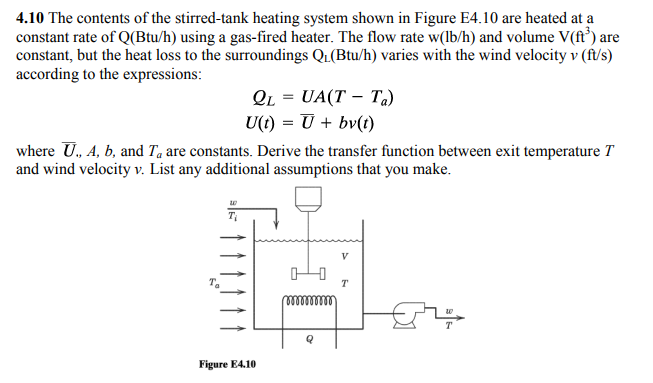4.10 The contents of the stirred-tank heating system shown in Figure E4.10 are heated at aconstant rate of Q(Btu/h) using a gas-fired heater. The flow rate w(lb/h) and volume V(ft') areconstant, but the heat loss to the surroundings Q1(Btu/h) varies with the wind velocity v (ft/s)according to the expressions:Q1 = UA(T-T.)where U, A, b, and Ta are constants. Derive the transfer function between exit temperature Tand wind velocity v. List any additional assumptions that you make.Figure E4.10

Questionhelp_outlineImage Transcriptionclose4.10 The contents of the stirred-tank heating system shown in Figure E4.10 are heated at a constant rate of Q(Btu/h) using a gas-fired heater. The flow rate w(lb/h) and volume V(ft') are constant, but the heat loss to the surroundings Q1(Btu/h) varies with the wind velocity v (ft/s) according to the expressions: Q1 = UA(T-T.) where U, A, b, and Ta are constants. Derive the transfer function between exit temperature T and wind velocity v. List any additional assumptions that you make. Figure E4.10 fullscreen
Step 1

Given that

Q(Btu/hr)= Constant rate of heat supply to the stirred tank heating system

W(lb/h)= flow rate of stream enter into the tank and is constant

V=volume of the tank and is constant

v= wind velocity

QL= heat loss to the surroundings= UA(Ti-T)

Assumptions:

1. There is perfect mixing in the tank
2. Density and specific heat both are constants
3. Temperature of inlet stream Ti is also constant.

Applying energy balance in the tank

Step 2

At steady state and assumed and given constants (U, b,A, Ta,Ti)

Step 3

Subtract 1 fr...

Want to see the full answer?

See Solution

Want to see this answer and more?

Our solutions are written by experts, many with advanced degrees, and available 24/7

See Solution
Tagged in

Chemical Engineering